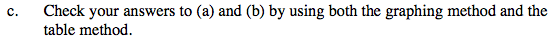### Home > PC > Chapter 8 > Lesson 8.1.3 > Problem8-43

8-43.
1. Let g(x) =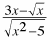. This is the same function you used in the previous problem. Homework Help ✎

1. Use the previous result, but not your calculator, to find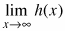where h(x) = 2 + g(x).

2.Without a calculator find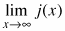where j(x) = log(g(x)).

3. Check your answers to (a) and (b) by using both the graphing method and the table method.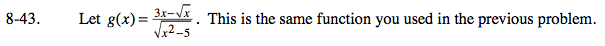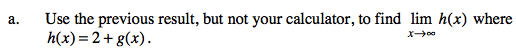The limit is 2 more than the limit for g(x).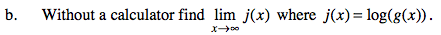log 3